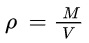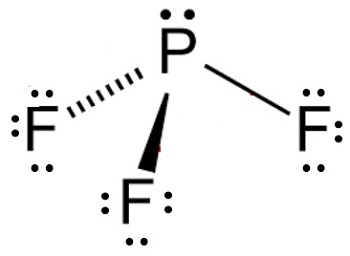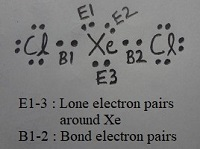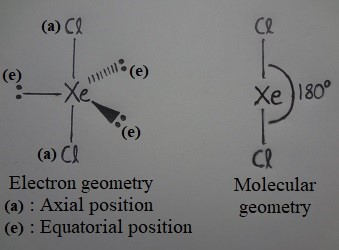# Top 15 Examination Question And Answer On Chemistry For Secondary School Students

Chemistry is the scientific discipline involved with compounds composed of atoms, i.e. elements, and molecules, i.e. combinations of atoms: their composition, structure, properties, behavior and the changes they undergo during a reaction with other compounds.Chemistry.In this article, we will examine the top 15 examination question and answer on chemistry for secondary school students.

## 1.Question:

Eugen Goldstein discovered in 1886 that atoms also have?

Eugen Goldstein discovered in 1886 that atoms also have a positive charge. He used a perforated cathode and detected positive rays, which he called anode rays or canal rays.

Ten years later J.J. Thomson discovered cathode rays, which are electrons. Ernest Rutherford is credited with discovering the positive rays that Goldstein observed were subatomic particles, which were named protons. This discovery happened in the gold foil experiment where helium nuclei were fired at a piece of gold foil. The positively charged helium nuclei bounced off of the positively charged gold nuclei where its protons were located.

## 2.Question:

How many valence electrons does chromium have?

Chromium has six valence electrons.

There are 5 electrons in the 3d shell and 1 electron in the 4s shell, we can conclude that there is a total of 6 valence electrons for chromium. The electron configuration of chromium is (Ar)4s13d5.

## 3.Question:

What is propane’s condensed structural formula?

Propane’s condensed structural formula is CH3 CH2 CH3. The prefix pro- means three and corresponds to three the three carbon atoms in propane’s formula. Each carbon has four bonds. So, the first carbon and last carbon have three bonds to hydrogen atoms and one bond to an adjacent carbon. The middle carbon is bound to two carbons, so it only has bonded to two hydrogen atoms.

## 4.Question:

What is the density of carbon dioxide at STP?

The density of carbon dioxide at STP is 0.00196 g/mL.

Density has the equation:• ρ is density
• m is mass
• v is volume

## 5.Question:

Is CH4 an ionic or covalent bond?

The bonds within the compound CH4 are covalent bonds.

Covalent bonds occur when atoms share outer electrons. Each carbon atom has four unpaired…

## 6.Question:

Where is wasabi on the Scoville scale?

Wasabi is not assessed using the Scoville scale. The scale measures the amount of ‘capsaicinoids,’ a chemical that causes pungency or heat, in food. Wasabi doesn’t have any capsaicinoids in it.

## 7.Question:

What is the conjugate base of H2 PO4 -?

The conjugate base of H2 PO4 – is HPO4 -2. The removal of a proton (H+1) from a conjugate acid gives us its conjugate base. Removing a +1 charge from the conjugate acid lowers the oxidation state by 1. HPO4 -2 is named hydrogen phosphate.

## 8.Question:

What is the chemical name for HCH3 CO2?

The chemical name for HCH3 CO2 is acetic acid.

Nomenclature rules for the combination of positive and negative ions lead us to the name hydrogen acetate, but this is an acid. In water, the hydrogen and acetate ions separate forming an acetic acid solution.

## 9.Question:

How does ATP supply energy for cellular activities?

ATP (adenosine tri-phosphate) supplies energy for cellular activities when one of its phosphate bonds is broken.

ATP has three phosphate bonds, and energy is stored in these bonds. When the cell needs energy, it breaks a phosphate bond, which releases the energy stored there. The cell can then use that energy to conduct its regular activities. The cell is then left with ADP (adenosine diphosphate), which has only two phosphate bonds. ADP is used in the cellular respiration reactions to create more ATP.

## 10.Question:

What are the building blocks of lipids?

The building blocks of lipids are one glycerol molecule and at least one fatty acid, with a maximum of three fatty acids.

Glycerol is a sugar alcohol with three OH groups. It acts as a backbone for fatty acids to bond.

Fatty acids are made up of a long hydrocarbon with a carboxyl group, which is represented as COOH. When the total number of carbons exceeds four, the carboxylic acid is known as a fatty acid.

To become a lipid, a combination must occur between fatty acids and glycerol called esterification. The reaction of glycerol and fatty acids forms a compound, which is lipids plus water. During the reaction, three water molecules are removed for them to combine.

Therefore, with one molecule of glycerol and at least one fatty acid, we will get the compound of lipids.

## 11.Question:

Is magnesium oxide ionic or covalent?

Magnesium oxide is considered ionic. This means that the bonds that hold magnesium and oxygen together are formed between atoms that give electrons or take electrons away from the other atoms.

In order to see how ionic bonds form, it is important to know the oxidation number of each of the atoms that are in the compound. Magnesium has an oxidation number of +2. This means that it wants to give two electrons away to be balanced. On the other hand, oxygen has an oxidation number of -2. This means that it needs 2 electrons to be balanced. When magnesium and oxygen are combined, they form a perfect pair, giving and taking the same amount of electrons.

## 12.Question:

What is the conjugate base of H2 CO3?

The conjugate base of H2 CO3 is HCO3 -.

To determine the conjugate base, remove a proton (H+) from the acid. The formula will have one less hydrogen atom and the charge will be reduced by 1.

## 13.Question:

What is the molecular geometry of PF3?

PF3 has a trigonal pyramidal molecular geometry.

The Lewis structure of PF3 is:Let’s count the areas around the phosphorus atom that contain at least two electrons. We see there are four. One of these pairs of electrons is not in a bond. The VSEPR theory tells us that when there are four areas of electron density, and where one includes an unshared pair of electrons, we have a trigonal pyramidal molecular geometry.

## 14.Question:

How many unpaired electrons does oxygen have?

Oxygen has two unpaired electrons.

Electrons in an atom can be identified in the shells. The maximum number of electrons in a shell depends on the formula 2n2, where n is the number of the shell. Each shell has orbitals, known as s, p, d and f. The first shell has one orbital, s, while the second shell has two orbitals, s and p. The number of orbitals increases with the number of shells.

To get the number of unpaired electrons, we must see the electron configuration. Oxygen’s atomic number is 8, so it has eight protons and electrons. These eight electrons are distributed in the shells. The electron configuration is 1s2 2s2 2p4. The maximum number of electrons in the second shell is 2n2 = 2 * 22 = 8. In this case, total electrons in the second shell = 2 (in s orbital) + 4 (in p orbital) = 6. So, the number of unpaired electrons = 8 – 6 = 2.

## 15.Question:

What is the value of the bond angle in XeCl2?

180° is the value of the bond angle in XeCl2.

The geometry of a covalent molecule can be predicted using valence shell electron pair repulsion theory (VSEPR theory). The Lewis dot structure of XeCl2 reveals three pairs of non-binding, or lone electrons, and two pairs of bond electrons around Xe. That means there are five electron pairs in the valence shell of Xe.According to VSEPR theory, when the total number of valence shell electron pairs around the central atom is five, the arrangement of electron pairs, or electron geometry, around the central atom is trigonal bipyramidal. Lone pairs of electrons around the central atom prefer equatorial positions to minimize repulsion.

In the case of XeCl2, there are three lone electron pairs around Xe that occupy equatorial positions, and therefore the two bond pairs (and hence the Cl atoms) occupy axial positions. The line passing through the Cl and Xe atoms is perpendicular to the equatorial plane containing the three lone electron pairs and the central atom Xe. Thus, the molecular geometry of XeCl2 is linear with a bond angle of 180°.